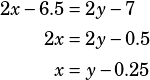Test 3 Section 4 #30

This one is yucky. Let’s attack it by substituting. First, we’re told that, so let’s reflect that (I’m going to use the decimal version offor simplicity):Now, let’s solve both equations for c:Because we now have two different equations with c isolated, we can substitute again here to get an equation that only contains xs and ys.That translates to “x is y minus,” so the answer is A.